# Design and comparison of MPPT based oscillatory wind turbine with conventional wind turbine

Design and comparison of MPPT based oscillatory wind turbine with conventional wind turbine

Divya ManukondaSrinivasa Rao Gorantla

Department of Electrical and Electronics Engineering, VFSTR University, Vadlamudi, Guntur district, Andhra pradesh, India

Corresponding Author Email:
Page:
589-600
|
DOI:
https://doi.org/10.3166/EJEE.20.589-600
|
Accepted:
|
Published:
31 December 2018
| Citation

OPEN ACCESS

Abstract:

The advantages of renewable energy resources encourage to implement an alternative for clean power generation. This paper presents design of a new oscillatory wind turbine which works on the principle of vortex vibrations. The power extracted from the oscillations is fed to Maximum power point tracking (MPPT) with fuzzy proportional derivative integral (PID) controller to track maximum power all the times. The resultant duty cycle of the MPPT with fuzzy PID controller is used for switching operations of high gain step up converter to boost up the voltage. A three level inverter has been used for DC-AC power conversion and the obtained electrical energy can be used for residential and commercial applications. In this paper a comparison has been made between power obtained from the conventional horizontal axis wind turbine, oscillatory wind turbine without MPPT & with MPPT for different wind speeds. The designing of the energy system and the results are verified using MATLAB simulink

Keywords:

oscillatory wind turbine, perturb and observe maximum power point tracking (MPPT), fuzzy PID controller, conventional wind Turbine

1. Introduction

Now a days there is a huge growth in power consumption, leading to diminution of conventional sources and increase in the pollution around the world. So a solution is required which provides power unlimitedly and leading to clean power production results in usage of renewable energy sources. As it is known there are number of renewable energy sources like wind, solar, tidal, biomass, etc., wind has advantages like clean, unlimited, environmental friendly and so wind is considered as a source for power production. Conventional horizontal axis wind turbine has been in usage for a number of years but it is not around the world because of huge investment, higher wind speed requirement, larger space requirement. So a system using wind advantages, eradicating its disadvantages has to be implemented.

Vortex based structural vibrations (Manfrida et al., 2016; Heinz et al., 2016; Ruscheweyh, 1994) is one of the new technology in which a structure vibrates with the passage of wind and these vibrations or oscillations of the structure can be converted to required form of energy. Among the number of oscillatory energy conversion devices like piezo electric cells, linear alternator, crack and pinion mechanism, an AC transducer (Baidwan and Kumar, 2015) has advantages like low cost, high power conversion, accuracy. As wind conditions (Wang, 2013; Cannistraro et al., 2017) vary all the times and so to utilize the power all the times battery has been used for storage. To achieve maximum power for varying wind conditions an MPPT Controller (Ali, 2016) can be used. But to achieve fast dynamic response fuzzy PID technique (Arulmurugan and Vanitha, 2013; Swain et al., 2016; Bouchakour et al., 2015) is attached to MPPT controller .The energy obtained from the oscillatory system may not be sufficient to run AC loads and to boost up the voltage to the required value high gain step up converter (Shuklal and Shrivastava, 2015) has been used in place of conventional boost converter having its own disadvantage like higher duty cycle requirement for larger voltage boosting. This boosted DC power can be converted to AC power using conventional inverter but it has disadvantages like larger THD, higher filter value requirement and so a three level inverter (Joshi and Agrawal, 2014) with less number of switches has been used.

The paper is organized as follows; the first section of the paper is dealing with the explanation of proposed oscillatory wind turbine. The second section explains MPPT with fuzzy PID controller and the third part discusses with the software realization of proposed system, results and discussion of the proposed system and the fourth part deals with the conclusion.

2. Proposed oscillatory wind turbine

The proposed oscillatory wind turbine which is shown in figure 1 works on the phenomenon of vortex based structural vibrations where the structure can be of any shape which is considered as tapered cylindrical structure because of symmetrical axis resulting in larger oscillations.

## 1.jpgFigure 1. Block diagram of proposed oscillatory wind turbine

When wind passes over the cylindrical structure vibrations are induced within the body and alternating lift forces (Manfrida et al., 2016) are developed around the structure which can be given as

${{F}_{Lift}}=0.5\rho {{U}^{2}}{{A}_{S}}{{C}_{Lift}}\sin ({{w}_{v}}t+\Phi )$   (1)

These alternating lift forces around the structure causes the structure oscillate and the resultant displacement of the structure can be given as

$X=\frac{{{F}_{Lift}}-m\ddot{x}}{K}$   (2)

A rod is placed within the structure attached to the transducer. Transducer is identical to the transformer but with two secondary windings consisting of magnetic core displaces with the structure and energy is induced in the two secondary windings.

Where X is the displacement,

is the wind speed, K is spring stiffness,  is the phase angle between structure and wind direction.

The resultant energy out of the transducer (Baidwan and Kumar, 2015) can be expressed as

${{V}_{outAC}}=\frac{{{V}_{inAC}}({{T}_{\sec }}C({{L}_{S}}^{2}-{{L}_{P}}^{2}))}{(4{{b}_{s}}{{T}_{pri}}{{C}_{T}})}$    (3)

This AC energy is converted into DC using diode bridge rectifier and fed to the battery for storage. But for general usage this energy is not sufficient and so high step up boost converter is used to boost up the converted oscillatory energy and the related expression can be

$\frac{{{V}_{outDC}}}{{{V}_{inDC}}}=\frac{3+K}{2-2K}$   (4)

This DC energy is converted to AC using three level inverter and can be used for residential and commercial applications.

Where

is the number of turns of secondary winding,  is the number of turns of primary winding,  is the total magnetic conductance.

3. MPPT controller

As it is known that wind is not constant and so to constant power may not be obtained from the system all the times. So a controller which tracks the maximum power has to be designed which is Perturb & observe maximum power point tracker. But at the same time fast and dynamic response is also required and so fuzzy with Proportional integral derivative controller (PID) is attached to the MPPT controller which continuously tracks maximum power based on all the environmental conditions.

The proposed MPPT with fuzzy PID controller is shown in below figure 2.

## 2.jpgFigure 2. Block diagram of MPPT with fuzzy PID controller

The DC values of voltage and current are collected from the diode bridge rectifier, fed to the controller and the resultant generated duty cycle is fed to the high gain step up converter. The flow chart explaining the step by step operation of the MPPT with fuzzy PID controller is shown in below figure 3.

## 3.jpgFigure 3. Block Diagram of MPPT with Fuzzy PID controller

The operation of MPPT with Fuzzy PID controller is explained in the following steps

i.) Read DC values of voltage and current from the diode bridge rectifier and fed to the perturb and observe tracker which tracks the maximum power until it is obtained with a number of perturbations and with a particular step size and the resultant is maximum voltage

which is maintained maximum at all the wind conditions.

ii.) This

is compared with the voltage from the diode bridge rectifier and the error is fed to fuzzy PID controller which processes the error with fuzzification and defuzzification.

iii.) This processed error is compared with the carrier and generates duty cycle for high gain step up converter.

The following are the fuzzy member ship functions for input and output in which seven membership functions are considered for the two inputs and rule base was constructed based on the membership functions and respective output membership functions are generated.

## 4.pngFigure 4. Input-1 membership function

## 5.pngFigure 5. Input-2 membership function

## 6.png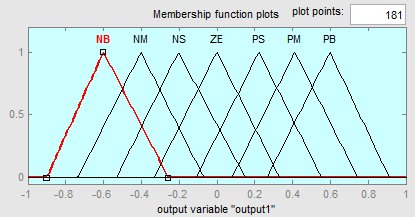Figure 6. Output membership function

4. Results and discussion

The proposed oscillatory wind turbine is simulated using MATLAB simulink and the following are the obtained results.

When the structure is passed by the wind due to vortex action it displaces and the value of displacement done by the structure is given in figure 7 for different wind speeds as 05m/s,1m/s,1.5m/s,2m/s where 2m/s is considered as running wind speed.

## 7.png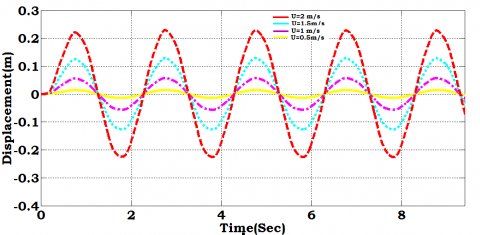Figure 7. Displacement made by the structure for different wind speeds

## 8.pngFigure 8. Output voltage of transducer

The above figure 8 shows the voltage obtained from AC transducer which is 52V AC.

## 9.pngFigure 9. Output voltage of diode bridge rectifier

The above figure 9 shows the voltage obtained from Diode Bridge Rectifier which is 49V DC.

## 10.pngFigure 10. Output voltage of high gain step up converter with out MPPT

The above figure 10 shows the voltage obtained from high gain DC-DC converter which is 230V DC without MPPT for different wind speeds.

## 11.pngFigure 11. Output current of high gain step up converter with out MPPT

The above figure 11 shows the current obtained from high gain DC-DC converter which is 4.5A without MPPT for working wind speed.

## 12.png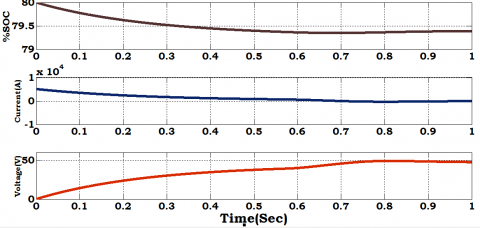Figure 12. Battery SOC, voltage and current

The above figure 12 shows the battery voltage current and % SOC.

## 13.pngFigure 13. Power of high gain step up converter without MPPT

The above figure 13 shows the voltage obtained from high gain DC-DC converter which is 1080W without MPPT for different wind speeds.

The below figure 14 shows the voltage obtained from high gain DC-DC converter which is 360V DC with MPPT for working wind speed.

## 14.pngFigure 14. Output voltage of high gain step up converter with MPPT

## 15.png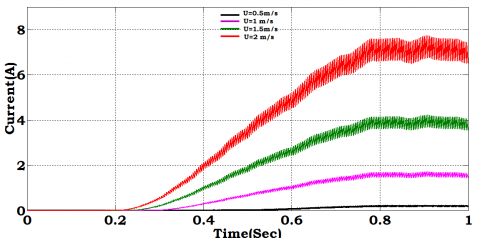Figure 15. Output current of high gain step up converter with MPPT

The above figure 15 shows the current obtained from high gain DC-DC converter which is 6.8A with MPPT for working wind speed.

## 16.png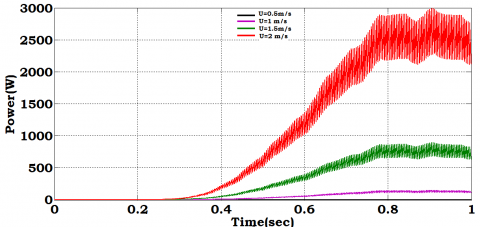Figure 16. Power of high gain step up converter with MPPT

The above figure 16 shows the power obtained from high gain DC-DC converter which is 2600W with MPPT for working wind speed.

Table 1. Comparison between conventional wind turbine and proposed oscillatory wind turbine with MPPT

 Wind speed (m/s) Proposed oscillatory wind turbine Conventional wind turbine with MPPT Without MPPT Fuzzy MPPT 0.5 V 4.88V 10V 1.225V I 0.1A 0.2A 0.001A P 0.5W 2W 0.001255W 1 V 51.84V 73V 1.3V I 1.037 A 1.5A 0.0018A P 52W 110W 0.00234W 1.5 V 130.1V 185V 1.6V I 2.6A 3.7A 0.0021A P 338W 685W 0.00336W 2.0 V 230V 350V 2V I 4.2A 6.8A 0.0025A P 1000W 2300W 0.0052W

The above table 1 shows the voltage, current, power of conventional horizontal axis wind turbine with MPPT, proposed oscillatory wind and without MPPT which shows that proposed oscillatory wind turbine works with lower wind speed and produces higher power when compared to the conventional wind turbine with lower wind speed.

Table 2. Specifications of the proposed oscillatory wind turbine

 S.No. Parameter Value 1. Length of the structure 2m 2. Inner diameter of structure 100mm 3. outer diameter of structure 200mm 4. Reynolds number 2,45,000 5. Strouhal number 0.2 6. Natural frequency 3Hz 7. Input voltage of AC transducer 5Vrms 8. Battery type Lead acid 9. Battery capacity 50Ah 10. Battery voltage 48V 11. Working wind speed 2m/s

The above table 2 shows the specifications used for designing oscillatory wind turbine.

5. Conclusions

An oscillatory wind turbine has been designed using MATLAB simulink and an MPPT based fuzzy PID controller has been used for generating pulse to high gain step up converter. The proposed wind turbine is compared with conventional wind turbine which shows that proposed oscillatory wind turbine with MPPT based Fuzzy PID controller generates high power when compared to conventional wind turbine with MPPT controller at lower wind speeds and results are verified using MATLAB simulink.

References

Ali U. S. (2016). Z-source DC-DC converter with fuzzy logic mppt control for photovoltaic applications. Energy Procedia, Vol. 90, pp. 163-170. https://doi.org/10.1016/j.egypro.2016.11.181

Arulmurugan R., Vanitha N. S. (2013), Intelligent fuzzy MPPT controller using analysis of DC to DC novel buck converter for photovoltaic energy system applications. 2013 International Conference on Pattern Recognition, Informatics and Mobile Engineering, pp. 225-231. https://doi.org/10.1109/ICPRIME.2013.6496477

Baidwan K. I. S., Kumar C. R. S. (2015). Design of linear variable differential transformer (LVDT) based displacement sensor with wider linear range characteristics. International Journal of Science & Technoledge, Vol. 3, No. 4, pp. 74-79.

Bouchakour A., Zaghba L., Brahami M., Borni A. (2015). Study of a photovoltaic system using MPPT buck-boost converter. International Journal of Materials, Mechanics and Manufacturing, Vol. 3, No. 1, pp. 65-68. https://doi.org/10.7763/ijmmm.2015.v3.168

Cannistraro G., Cannistraro M., Trovato G. (2017). Islands "Smart Energy" for eco-sustainable energy a case study "Favignana Island". International Journal of Heat and Technology, Vol. 35, No. 1, pp. S87-S95. https://doi.org/10.18280/ijht.35Sp0112

Heinz J. C., Sørensen N. N., Zahle F., Skrzypiński W. (2016). Vortex-induced vibrations on a modern wind turbine blade. Wind Energy, Vol. 19, No. 11, pp. 2041-2051. https://doi.org/10.1002/we.1967

Joshi H., Agrawal R. (2014). Topologies of multilevel inverter-a review. World Academics Journal of Engineering Sciences, Vol. 1, No. 1, pp. 1009-1-1009-7. https://doi.org/10.15449/wjes.2014.1009

Manfrida G., Rinchi M., Soldi G. (2016). Dynamic model of a vortex-induced energy converter. Journal of Energy Resources Technology, Vol. 138, No. 6. https://doi.org/10.1115/1.4033587

Ruscheweyh H. (1994). Vortex excited vibrations. Wind-Excited Vibrations of Structures, Vol. 335, pp. 51-84. https://doi.org/10.1007/978-3-7091-2708-7_2

Shuklal J., Shrivastava J. (2015). Analysis of PV array system with buck-boost converter using perturb & observe method. International Journal of Innovative Research in Electrical, Electronics, Instrumentation and Control Engineering, Vol. 3, No. 3, pp. 51-57. https://doi.org/10.17148/IJIREEICE.2015.3311

Swain N., Panigrahi C. K., Pati N. (2016). Comparative performance analysis of dc-dc converter using PI controller and fuzzy logic controller. IEEE 1st International Conference on Power Electronics, Intelligent Control and Energy Systems (ICPEICES), pp. 1-5. https://doi.org/10.1109/ICPEICES.2016.7853232

Wang G. (2013). Design and simulation of stand-alone wind-solar hybrid generating system. Applied Mechanics and Materials, Vol. 260-261, pp. 224-230. https://doi.org/10.4028/www.scientific.net/AMM.260-261.224